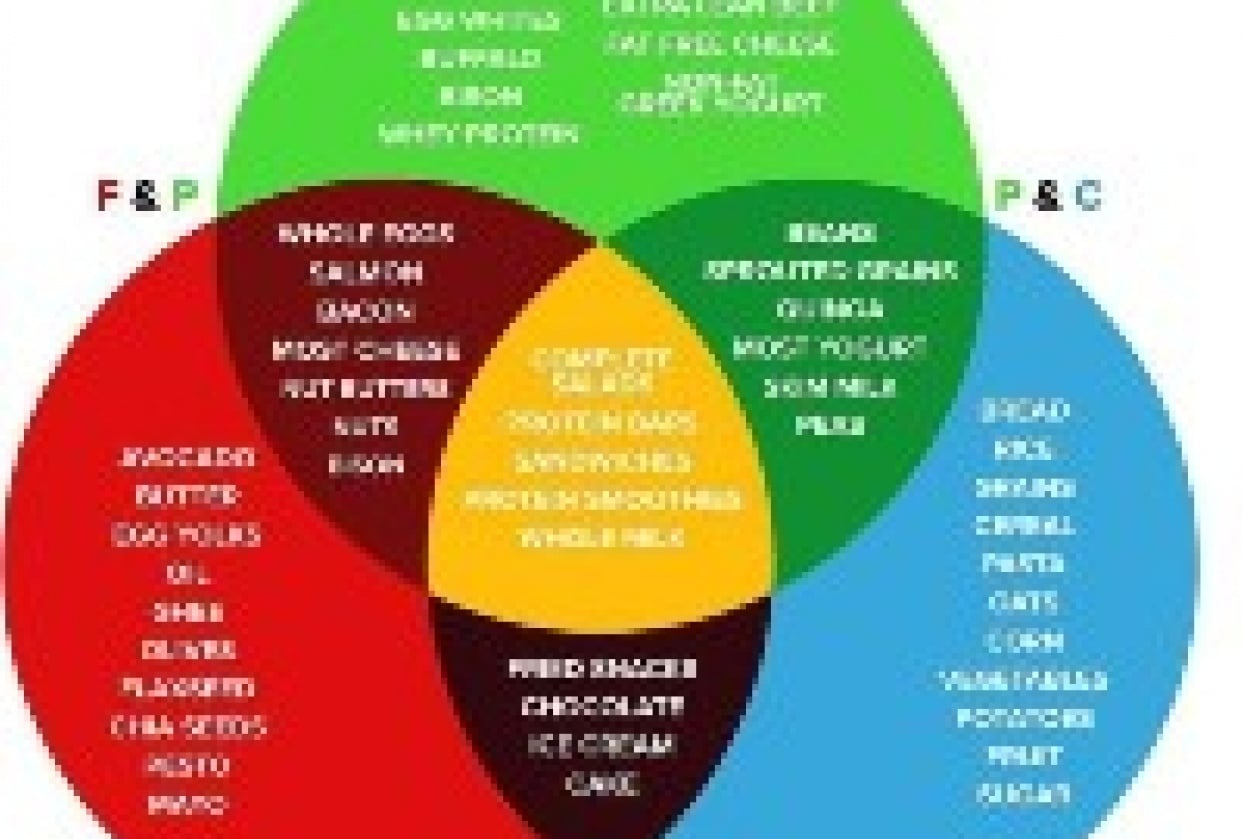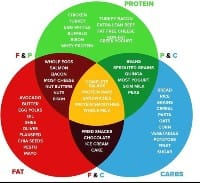## Create My PlanCreate My Plan

Protein = 0.8g x 227lbs (body weight) = 182g / 728 cal (182 x 4 = 728)

Carbs = 1.25g x 227lbs (body weight) = 284g / 1136 cal (284 x 4 = 1136)

Fat = 0.3g x 154lbs (fat free body weight) = 46g / 414 cal (34 x 9 = 414)

Total daily calories = 2,278 calories

for fat loss drop 20% therefore 2278 x 20% = 455

so daily calories for fat loss will be 2278 - 455 = 1823 calories

Protein now 583 cals / 146g

Carbs now 909 cals / 227g

Fat now 331 cals / 37g